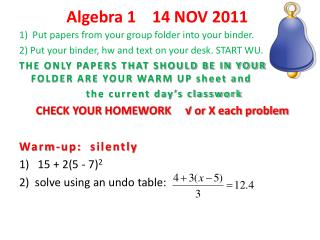# Algebra 1 14 NOV 2011 - PowerPoint PPT PresentationDownload PresentationAlgebra 1 14 NOV 2011

Algebra 1 14 NOV 2011Download Presentation## Algebra 1 14 NOV 2011

- - - - - - - - - - - - - - - - - - - - - - - - - - - E N D - - - - - - - - - - - - - - - - - - - - - - - - - - -
##### Presentation Transcript

1. Algebra 1 14 NOV 2011 1) Put papers from your group folder into your binder. 2) Put your binder, hwand text on your desk. START WU. THE ONLY PAPERS THAT SHOULD BE IN YOURFOLDER ARE YOUR WARM UP sheet and the current day’s classwork CHECK YOUR HOMEWORK √ or X each problem Warm-up: silently 1) 15 + 2(5 - 7)2 2) solve using an undo table:

2. Objective Students will write a linear equation given a recursive sequence, graph or data. Students will take notes, do an investigation and use think-pair-share.

3. Homework due today pg. 193: 11, 12 Homework due next class pg. 182: 1, 2, 4 study for test on Wed/ Thurs recursive rules using undo boxes order of operations situations, tables, graphs and rules slope, y-intercept, equations

4. debrief van, car, truck step 6) where does the starting value for each show up on the graph? LABEL EACH on your graph. step 7) Which line represents the minivan? How can you tell? step 8)Where are the vehicles when the minivan meets the car? the truck? MARK it on your graph. step 9) which vehicle is traveling faster- the sports car or truck? how can you tell? Draw an ARROW pointing to the line of the fastest vehicle on your graph. step 10) which vehicle arrives at its destination first? HOW CAN YOU TELL? BOX this on your graph.

5. Summary- graph from recursive rule Quantities generated by a recursive additive sequence have a LINEAR RELATIONSHIP because their graphs lie on a straight line. They have a CONSTANT RATE OF CHANGE, described in the recursive rule. If the amount added is positive, the graph rises from left to right (positive slope) If the amount added is negative, the graph falls from left to right (negative slope)

6. NOTES- finding equations Intercept form y = b + mx (OR y = mx + b ) of a linear function y = b ± mx y = b + mx Slope goes with the x 1. where does it start? y-intercept, value when x = 0 2. increasing or decreasing? 3. By how much? (add or subtract repeatedly x times)

7. Linear Equations and the Intercept Formpg. 178 section 3.4 Investigation “working out with equations” Steps 1 – 3 Read the steps Write what we KNOW and WANT to find Calculator routine {0,215} Enter {Ans(1)+1,Ans(2)+3.8}

8. investigation… Steps 4 – 7 Read carefully Write what you KNOW and WANT to find THINK– PAIR– SHARE Step 8– PLOT by hand on graph paper Do steps 9 and 10

9. pg. 181 example B Write what you KNOW and WANT to find for each part. REPEAT steps a - e for Sports Car— KNOW– starts 35 miles from Flint and travels away from Flint at 48 mi/h REPEAT steps a – e for Truck— KNOW- starts 0 miles from Flint and travels away from Flint at 66 mi/h

10. do pg. 182+ 2. graph on calculator and answer questions Y= 24 – 45x 3. graph on calculator and answer questions Y= 4.7 + 2.8x DO 5, 8, 9, 10, 14, 15

11. strategies? what strategies did you use to find the rule? what math did we do today? what was new? what was review?

12. Algebra 1 15 NOV 2011 CHECK YOUR HOMEWORK √ or X each problem PLEASE TURN IN HOMEWORK to the substitute. Warm-up: silently- write in your notes slope m = from a table- find the differences in y-values find the differences in x-values y-intercept -the y-value when x = 0 -on a graph- the y- value where the line crosses the y-axis

13. Objective Students will find slope and y-intercept from tables and graphs. Students will work with their group and present their work to the class using the doc-cam.

14. Homework due today pg. 182: 1, 2,4 Homework due next class WORKSHEET if not completed in class study for test recursive rules using undo boxes order of operation situations, tables, graphs and rules slope, y-intercept, equations

15. Algebra 1 16/17 NOV 2011 1) Put papers from your group folder into your binder. 2) Put your binder, hw and text on your desk. START WU. THE ONLY PAPERS THAT SHOULD BE IN YOURFOLDER ARE YOUR WARM UP sheet and the current day’s classwork CHECK YOUR HOMEWORK √ or X each problem Warm-up: silently USE PE M(D) A(S) work left to right! 1) 18 ÷3 x 2 – 10 + 32 2) 15 – 16 ÷ 2 + 2(5-3) + 10

16. Objective Students will show proficiency on unit ideas. Students will take a written test.

17. Homework due today worksheet Homework due next class pg. 209: 15, 16

18. TEST- Do your best! Silence please. When you are finished, you may silently work on your homework.